# Did amphetamines help Erdős?

During my work on the Paris talk I began to wonder whether Paul Erdős (who I used as an example of a respected academic who used cognitive enhancers) could actually have been shown to have benefited from his amphetamine use, which began in 1971 according to Hill (2004). One way of investigating is his publication record: how many papers did he produce per year before or after 1971? Here is a plot, based on Jerrold Grossman’s 2010 bibliography: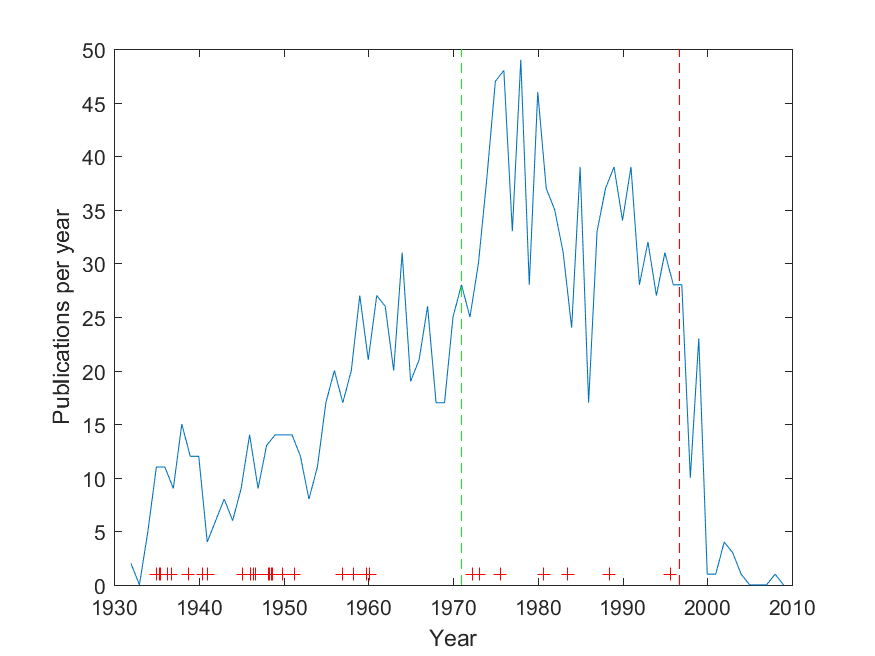Productivity of Paul Erdos over his life. Green dashed line: amphetamine use, red dashed line: death. Crosses mark named concepts.

The green dashed line is the start of amphetamine use, and the red dashed life is the date of death. Yes, there is a fairly significant posthumous tail: old mathematicians never die, they just asymptote towards zero. Overall, the later part is more productive per year than the early part (before 1971 the mean and standard deviation was 14.6±7.5, after 24.4±16.1; a Kruskal-Wallis test rejects that they are the same distribution, p=2.2e-10).

This does not prove anything. After all, his academic network was growing and he moved from topic to topic, so we cannot prove any causal effect of the amphetamine: for all we know, it might have been holding him back.

One possible argument might be that he did not do his best work on amphetamine. To check this, I took the Wikipedia article that lists things named after Erdős, and tried to find years for the discovery/conjecture. These are marked with red crosses in the diagram, slightly jittered. We can see a few clusters that may correspond to creative periods: one in 35-41, one in 46-51, one in 56-60. After 1970 the distribution was more even and sparse. 76% of the most famous results were done before 1971; given that this is 60% of the entire career it does not look that unlikely to be due to chance (a binomial test gives p=0.06).

Again this does not prove anything. Maybe mathematics really is a young man’s game, and we should expect key results early. There may also have been more time to recognize and name results from the earlier career.

In the end, this is merely a statistical anecdote. It does show that one can be a productive, well-renowned (if eccentric) academic while on enhancers for a long time. But given the N=1, firm conclusions or advice are hard to draw.

## Shakespeare is common

One way of seeing that Shakespearian numbers are the generic case is to imagine choosing a number randomly. It has probability$S$ of being in the level 1 interval of Shakespearian numbers. If not, then it will be in one of the 9 intervals 1/10 long that don’t start with the correct first digit, where the probability of starting with Shakespeare in the second digit is$S$. If that was all there was, the total probability would be$S+(9/10)S+(9/10^2)S+\ldots = 10S<1$. But the 1/10 interval around the first Shakespearian interval also counts: a number that has the right first digit but wrong second digit can still be Shakespearian. So it will add probability.

Another way of thinking about it is just to look at the initial digits: the probability of starting with$[Shakespeare]$ is$S$, the probability of starting with$[Shakespeare]$ in position 2 is$(1-S)S$ (the first factor is the probability of not having Shakespeare first), and so on. So the total probability of finding Shakespeare is$S + (1-S)S + (1-S)^2S + (1-S)^3S + \ldots = S/(1-(1-S))=1$. So nearly all numbers are Shakespearian.

This might seem strange, since any number you are likely to mention is very likely Shakespeare-free. But this is just like the case of transcendental, normal or uncomputable numbers: they are actually the generic case in the reals, but most everyday numbers belong to the algebraic, non-normal and computable numbers.

It is also worth remembering that while all normal numbers are (almost surely) Shakespearian, there are non-normal Shakespearian numbers. For example, the fractional number$0.[Shakespeare]000\ldots$ is non-normal but Shakespearian. So is$0.[Shakespeare][Shakespeare][Shakespeare]\ldots$ We can throw in arbitrary finite sequences of digits between the Shakespeares, biasing numbers as close or far as we want from normality. There is a number$0.[Shakespeare]3141592\ldots$ that has the digits of$\pi$ plus Shakespeare. And there is a number that looks like$\pi$ until Graham’s number digits, then has a single Shakespeare and then continues. Shakespeare can hide anywhere.

In things of great receipt with case we prove,
Among a number one is reckoned none.
Then in the number let me pass untold,
Though in thy store’s account I one must be
-Sonnet 136

# Rational fractal distributionsMost of the time we encounter probability distributions over the reals, the positive reals, or integers. But one can use the rational numbers as a probability space too.

Recently I found the paper Vladimir Trifonov, Laura Pasqualucci, Riccardo Dalla-Favera & Raul Rabadan. Fractal-like Distributions over the Rational Numbers in High-throughput Biological and Clinical Data. Scientific Reports 1:191 DOI: 10.1038/srep00191. They discuss the distribution of ratios of the number of reads from the same spot of DNA that come from each chromosome in a pair: the number of reads is an integer, so the ratio is rational. They get a peaky, self-similar distribution empirically, and the paper explains why.

If you take positive independent integers from some distribution$f(n)$ and generate ratios$q=a/(a+b)$, then those ratios will have a distribution that is a convolution over the rational numbers:$g(q) = g(a/(a+b)) = \sum_{m=0}^\infty \sum_{n=0}^\infty f(m) g(n) \delta \left(\frac{a}{a+b} - \frac{m}{m+n} \right ) = \sum_{t=0}^\infty f(ta)f(tb)$

One can of course do the same for non-independent and different distributions of the integers. Oh, and by the way: this whole thing has little to do with ratio distributions (alias slash distributions), which is what happens in the real case.

The authors found closed form solutions for integers distributed as a power-law with an exponential cut-off and for the uniform distribution; unfortunately the really interesting case, the Poisson distribution, doesn’t seem to have a neat closed form solution.

In the case of a uniform distributions on the set$\{1,2,\ldots , L\}$ they get$g(a/(a+b)) = (1/L^2) \lfloor L/\max(a,b) \rfloor$.

They note that this is similar to Thomae’s function, a somewhat well-known (and multiply named) counterexample in real analysis. That function is defined as f(p/q)=1/q (where the fraction is in lowest terms). In fact, both graphs have the same fractal dimension of 1.5.

It is easy to generate other rational distributions this way. Using a power law as an input produces a sparser pattern, since the integers going into the ratio tend to be small numbers, putting more probability at simple ratios:

If we use exponential distributions the pattern is fairly similar, but we can of course change the exponent to get something that ranges over a lot of numbers, putting more probability at nonsimple ratios$p/q$ where$p+q \gg 1$: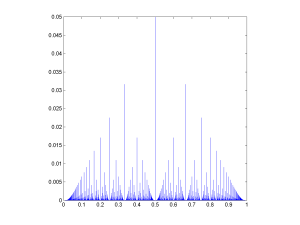The rational distribution of two convolved Exp[0.1] distributions.Not everything has to be neat and symmetric. Taking the ratio of two unequal Poisson distributions can produce a rather appealing pattern: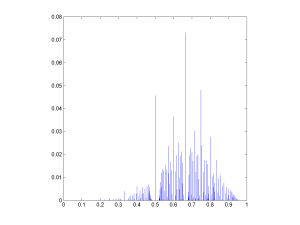Rational distribution of ratio between a Poisson and a Poisson variable.Of course, full generality would include ratios of non-positive numbers. Taking ratios of normal variates rounded to the nearest integer produces a fairly sparse distribution since high numerators or denominators are rare.

But multiplying the variates by 10 produces a nice distribution.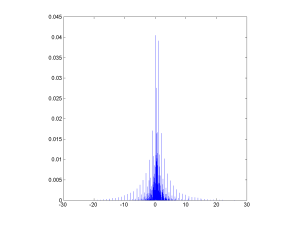Rational distribution of a/(a+b) ratios of normal variates that have been multiplied by 10 and rounded.

This approaches the Chauchy distribution as the discretisation gets finer. But note the fun microstructure (very visible in the Poisson case above too), where each peak at a simple ratio is surrounded by a “moat” of low probability. This is reminiscent of the behaviour of roots of random polynomials with integer coefficients (see also John Baez page on the topic).

The rational numbers do tend to induce a fractal recursive structure on things, since most measures on them will tend to put more mass at simple ratios than at complex ratios, but when plotting the value of the ratio everything gets neatly folded together. The lower approximability of numbers near the simple ratios produce moats. Which also suggests a question to ponder further: what role does the über-unapproximable golden ratio have in distributions like these?

# Mathematical anti-beauty

Browsing Mindfuck Math I came across a humorous Venn diagram originally from Spikedmath.com. It got me to look up the Borwein integral. Wow. A kind of mathematical anti-beauty.

“As we all know”,$sinc(x)=sin(x)/x$ for$x\neq 0$ and defined to be 1 for$x=0$. It is not that uncommon as a function. Now look at the following series of integrals:$\int_0^{\infty} sinc(x) dx = \pi/2$,$\int_0^{\infty} sinc(x) sinc(x/3) dx = \pi/2$ ,$\int_0^\infty sinc(x) sinc(x/3) sinc(x/5) dx = \pi/2$.

The pattern continues:$\int_0^\infty sinc(x) sinc(x/3) sinc(x/5) \cdots sinc(x/13) dx = \pi/2$.

And then…$\int_0^\infty sinc(x) sinc(x/3) sinc(x/5)$$\cdots sinc(x/13) sinc(x/15) dx$$=\frac{467807924713440738696537864469}{935615849440640907310521750000}\pi$

It is around 0.499999999992646$\pi$ – nearly a half, but not quite.

What is going on here? Borwein & Borwein give proofs, but they are not entirely transparent. Basically the reason is that 1/3+1/5+…1/13 < 1, while 1/3+1/5+…1/13 + 1/15 > 1, but why this changes things is clear as mud. Thankfully Hanspeter Schmid has a very intuitive explanation that makes what is going on possible to visualize. At least if you like convolutions.

In any case, there is something simultaneously ugly and exciting when neat patterns in math just ends for no apparent reason.

Another good example is the story of the Doomsday conjecture. Gwern tells the story well, based on Klarreich: a certain kind of object is found in dimension 2, 6, 14, 30 and 62… aha! They are conjectured to occur in all$2^n-2$ dimensions. A branch of math was built on this conjecture… and then the pattern failed in dimension 254. Oops.

It is a bit like the opposite case of the number of regular convex polytopes in different dimensions: 1, infinity, 5, 6, 3, 3, 3, 3… Here the series start out crazy, and then becomes very regular.

Another dimensional “imperfection” is the behaviour of the volume of the n-sphere:$V_n(r)=\frac{\pi^{n/2}r^n}{\Gamma(1+n/2)}$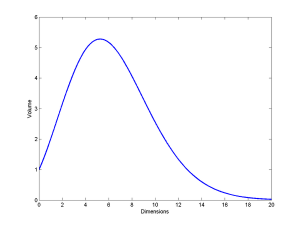The volume of a unit sphere increases with dimension until$n \approx 5.25$, and then decreases. Leaving the non-intuitiveness of why volumes would shrink aside, the real oddness is that the maximum is for a non-integer dimension. We might argue that the formula is needlessly general and only the integer values count, but many derivations naturally bring in the Gamma function and hence the possibility of non-integer values.

Another association is to this integral problem: given a set of integers$x_i$, is the integral$\int_0^\pi \prod_i \sin(x_i \theta) d\theta = 0$ ? As shown in Moore and Mertens, this is NP-complete. Here the strangeness is that integrals normally are pretty well behaved. It seems absurd that a particular not very scary trigonometric integral should require exponential work to analyze. But in fact, multivariate integrals are NP-hard to approximate, and calculating the volume of a n-dimensional polytope is actually #P-complete.

We tend to assume that mathematics is smoother and more regular than reality. Everything is regular and exceptionless because it is generated by universal rules… except when it isn’t. The rules often act as constraints, and when they do not mesh exactly odd things happen. Similarly we may assume that we know what problems are hard or not, but this is an intuition built in our own world rather than the world of mathematics. Finally, some mathematical truths maybe just are. As Gregory Chaitin has argued, some things in math are irreducible; there is no real reason (at least in the sense of a comprehensive explanation) for why they are true.

Mathematical anti-beauty can be very deep. Maybe it is like the insects, rot and other memento mori in classical still life paintings: a deviation from pleasantness and harmony that adds poignancy and a bit of drama. Or perhaps more accurately, it is wabi-sabi.